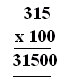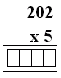Name: ___________________Date:___________________

 Email us to get an instant 20% discount on highly effective K-12 Math & English kwizNET Programs!

### Grade 3 - Mathematics9.10 Multiplication Review Test

 Q 1: In the multiplication problem shown what is the product?7936312567680 Q 2: 123 x 2 = Answer: Q 3: In the multiplication problem shown what are the fators?315100315 and 100 Q 4: 1675 x 5Answer: Q 5: In the multiplication problem shown what is the product?12036156 Q 6: Find the product of the multiplication.420242304200 Q 7: Find the product of the multiplication.100010101020 Q 8: Find the Product of the multiplication.Answer: Question 9: This question is available to subscribers only! Question 10: This question is available to subscribers only!